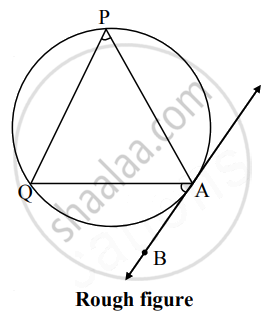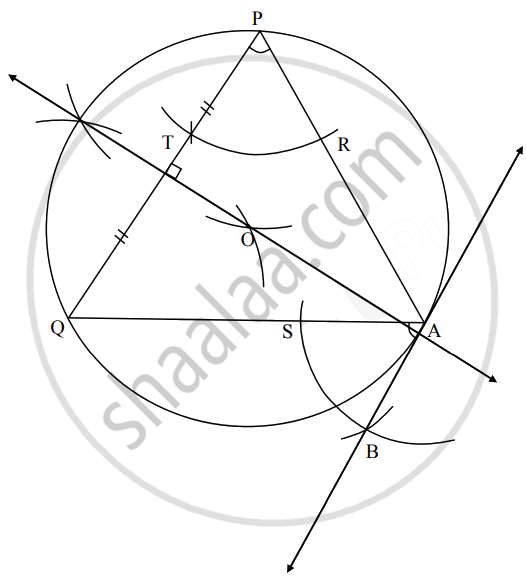# Take point P and Q and draw a circle passing through them. Draw a tangent AB to the circle without using the centre of the circle - Geometry

Diagram

Take point P and Q and draw a circle passing through them. Draw a tangent AB to the circle without using the centre of the circle.

#### SolutionAnalysis:

Centre of the circle passing through P and Q must be equidistant from points P and Q.

∴ It must lie of perpendicular bisector of seg PQ.Steps of construction:

1. Take any two point P, Q and join them.
2. Draw a perpendicular bisector of PQ.
3. Take any point O on the perpendicular bisector and draw a circle with centre O and radius OP.
4. Take any point A on the major arc of the circle and draw ∆PQA.
5. By taking P as centre and any convenient distance on compass draw an arc intersecting the arms of ∠QPA in points T and R.
6. With A as centre and the same distance in the compass, draw an arc intersecting the chord QA at point S.
7. Taking radius equal to TR and S as centre, draw an arc intersecting the previously drawn arc. Name the point of intersection as B.
8. Draw line AB. Line AB is the required tangent to the circle.
Concept: Construction of a Tangent to the Circle at a Point on the Circle
Is there an error in this question or solution?

Share